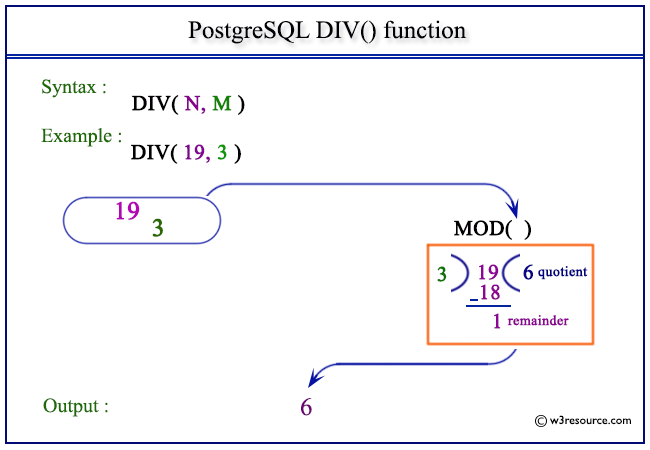# PostgreSQL DIV() function

## DIV() function

The PostgreSQL div() function is used to return the integer quotient of a division as specified in the argument.

Syntax:

```div()
```

PostgreSQL Version: 9.3

Pictorial presentation of PostgreSQL DIV() functionExample: PostgreSQL DIV() function

Code:

``````SELECT DIV(19,3) AS "Quotient";
```
```

Sample Output:

``` Quotient
----------
6
(1 row)
```

Example: PostgreSQL DIV() function using negative value

Code:

``````SELECT DIV(-27,5) AS "Quotient";
```
```

Sample Output:

``` Quotient
----------
-5
(1 row)ssss
```

Previous: DEGREES function
Next: EXP function

﻿